Maths-
General
Easy

Question

# If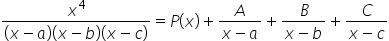then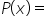##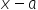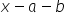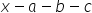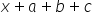Hint:

## The correct answer is:### Given :To Find : P(x)Let P(x) be a linear polynomial i.e.  = mx + n ( since y = mx + c)Substitute this value in the equation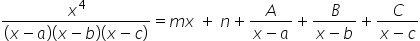Taking LCM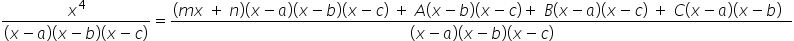Cancelling denominators on both sides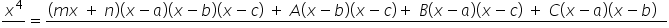On comparing the coefficients of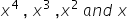from both sides ,we get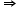m = 1-am -bm -cm + n = 0a + b + c = nP(x) =mx + nSubstituting the values of m and n inP(x) = x + a + b + c#### With Turito Foundation.#### Get an Expert Advice From Turito.